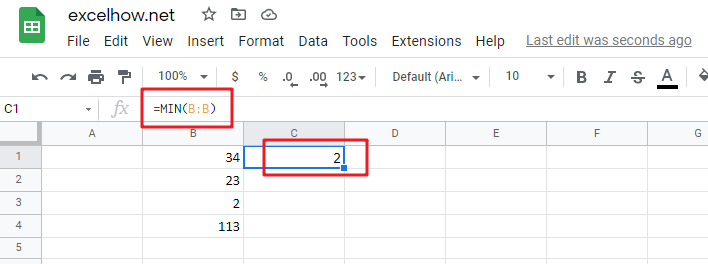# ExcelHow

This post will guide you how to use Google Sheets MIN function with syntax and examples in Google Spreadsheet.

## Description

The Google Sheets MIN function returns the smallest numeric value from the numbers that you provided. Or returns the smallest value in the array.

The MIN function is a build-in function in Google Sheets and it is categorized as a Statistical Function.

## Syntax

The syntax of the MIN function is as below:

= MIN(num1,[num2,…numn])

Where the MIN function arguments are:

• num1 -This is a required argument. A number, or a reference to a number or a range that contains numeric values
• Num2,…Numn – This is an optional argument.  A number, or a reference to a number or a range that contains numeric values

Note:

• The MIN function will not compare empty cells
• The MIN function can accept up to 255 arguments with numeric value.
• The MIN function will ignore Boolean values (TRUE and FALSE)
• The MIN function will ignore numbers that the format is text.
• The returned value of The MIN function is a numeric value.

## Google Sheets MIN Function Examples

The below examples will show you how to use Google Sheets MIN Function to get the smallest value form the numbers.

#1 To get the smallest value from the column A, just using the following Google Sheets formula:

`=MIN(B:B)`